﻿ Aplication of Piezoelectric Effects on Flutter Speed of Gas Turbine BladesPublications are Open
Access in this journal
Article Versions
Export Article
• Normal Style
• MLA Style
• APA Style
• Chicago Style
Research Article
Open Access Peer-reviewed

### Aplication of Piezoelectric Effects on Flutter Speed of Gas Turbine Blades

Ali Ghorbanpoor Arani, Seyed Adnan Mousavi
American Journal of Mechanical Engineering. 2017, 5(5), 223-227. DOI: 10.12691/ajme-5-5-5
Published online: November 16, 2017

### Abstract

Analysis of gas turbines blades is one of the important applications of aeroelastic. In this study, a row of blade including a tuned disk and a certain number of blades are studied. Curved blades are modeled as free beam whose section has a significant moment of inertia due to the curvature. The blades are under self-exited flutter with two degrees of bending and torsional vibration freedom. Since the disk is tuned, analysis of the entire structure and fluid system is focused on a blade and the flow around it. Aerodynamic forces are calculated with ANSYS/FLOTRAN CFD software following the stable and unstable blades steps and then, the real and imaginary forces of the fluid are obtained. Aerodynamic forces at different reduced frequencies are measured based on the above method. On the other hand, Timoshenko beam motion equation is written with regard to the rotary inertia and shear deformation. Ignored external forces and modal analysis while flexural and torsional movements are out of the coupled state , is used to determine the natural frequencies and modes of the system. Then, the final aero-elastic analysis is carried out in coupling flexural and torsional and coupling aerodynamic condition. By involvement of factors such as quasi –inertia, quasi- damping and quasi-elastic fluid in inertia and damping and stiffness matrix, and in the form of special value is created. Generally, the obtained special value will be a mixed number. If the release rate of the fluid is in a way that the real part of it which represents the damping rate, equals to zero, and such speed is the line of divergence and is called the flutter speed. In this case, the perceived part will represent the frequency of flutter. Then, the velocity of the flutter with the addition of piezoelectric is studied, piezoelectric effects on the velocity of the blades are investigated. At the end, flexural and torsional displacements of the blade have been analyzed over time.

### 1. Introduction

Unwanted break of gas turbine is a considerable problem in the turbinate because of the exorbitant financial costs and unexpected stop in production process. The main causes of blades breakage include vibration, fatigue, corrosion, erosion, and creep. Of these, we can say that the vibrations of the blades is the most complex issue. Blade vibration is often examined from two aspects: A) flutter B) mandatory response. These two factors lead to high cycle fatigue and reduced blade life. These two topics are different aspects of blades aeroelastic analysis. Aeroelastic is the study of static and dynamic behavior of structural elements in fluid flows. In other words, aeroelastic is an interactions between deformation of elastic structures in air flow and the resulting aerodynamic forces. In general, the progresses in calculations of aeroelastic blade vibration is in two directions. A) In terms of the status of blade structure (straight/curved blade and its thickness) and B) considering the flow conditions in terms of subsonic/ transonic/ultrasonic or compressibility. Numerous studies have been conducted so far for these two directions. Here the main developments of these methods and the names of the first theorists are mentioned.

### 2. Modal Analysis of Disk System and Blades

We know that the vibration of whole blade is the result of superposition of different temporal frequancies. In addition, the blade row has cyclic and axial symmetry. Because of Its linearity, blade vibration can be considered as the sum of the space waves. Phase differences of each blade from adjacent blade during oscillation in one of the space modes equal to the following amount:(1)

which is called Inter blade phase angle. In this equation, j is the number of nodal diameter and r is the total number of blades. Thus, other quantities of the flow comply the same relationship: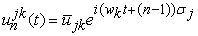(2)

Thus, according to the system's physical homogeneity, it is possible to calculate one of the blades and then calculate the movement of other blades between frequency and phase, using the above equation.

### 3. Structural Model

Blade oscillations have two cyclic(a) and torsional (h) directions. In these two directions, especially in the flexural, many in vacuum frequencies can be achieved. However, condition monitoring surveys show that due to high frequency of vibrations, the first frequency of the blades is their dominant phenomenon of aeroelastic vibrations. Therefore, this analysis has been investigated in the first mode with a plunge degree of freedom and a pitch degree of freedom. However, at first, it is necessary to calculate natural flexural and torsional frequency of beam.

### 4. Motion Equation of the Blade Using Timoshenko Beam Theory

If the thickness of the beam is also considered in calculation of its dynamic behavior, the resulting model is called Timoshenko beam. Rotary inertia, as well as shear deformation are also considered in these beams. Once the value of the kinetic and potential energy parameter are obtained, and the Lagrangian expression is placed in the Hamilton’s principle and become simplified, the following equation of motion is given(3)

And the boundary conditions of a cantilever beam are applied on it. In calculating the natural flexural frequency, airfoil mass center is the reference motion of the first and second couple.

### 5. Calculation of Natural Flexural Frequencies and Mode Shapes

Prior to determining the final coupled motion of the object, in order to find natural frequencies and mode, the external forces are put equal to zero and the flexural and torsional expressions are converted into uncoupled, so that natural flexural () and torsional (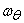) frequencies are achieved separately.

To do this, we convert the equation between the first and second relationship (3)to a special value problem.(4)

The above system of equation is a special value problem. As we can see, the equations have been written in dimensionless form and after required operations, the system has converted to a form in accordance with the provided relations and accordingly, the formula for calculation of natural frequency of flexural beam is obtained.

Here, methods to solve these equations is described according to the above relations with other aeroelastic analysis process and the use of this relations in computational planning. If we assume the dimensionless temporal frequency as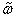and the spatial frequency as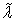then the issue of special value is based on Eq. 5 and solving is based on Eq.6.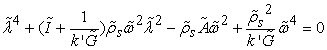(5)(6)

As we can see, the characteristic equation has four roots of which two roots are always imaginary and ifis less than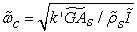both square roots are real and if it is more than square roots. The absolute value of the first two roots (a) and the two square roots (b) are called wave numbers.

### 6. Calculation of Natural Torsional Frequency and Mode Shape

After a simplifying, the third equation of beam motion, the torsional natural frequency, and normalizer mode shape is obtained.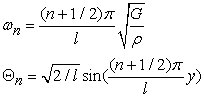(7)

### 7. Aerodynamic Model

Here, in order to obtain the airfoil surface pressure distribution, aerodynamic analysis is carried out using CFD and the capabilities of ANSYS/CFD/FLOTRAN. ANSYS is a product of Multi physics and one of its capabilities is to solve CFD of fluid systems by numerical FEM method. FLOTRAN has a subset of ALE that performs the interactions between fluid and structure in the motion boundaries between fluid and structure. Fluid boundaries include intermittent or periodic boundaries, torn levels and input and output of the fluid. This procedure was performed in Blades marching steps and boundary conditions between fluid structure was introduced to ANSYS according to Table 9.

### 8. Formation of Aeroelastic Equation

In order to analyze the flutter, equation (3) is written with mechanical and aeroelastic coupling. By having the natural flexural and torsional frequencies at hand, extractingandfrom Eq. 3, performing the necessary replacements, converting the parameters to the Rayleigh-Ritz form, multiplying the first equation in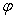, second equation inand third equation inand taking integral with respect to y in blade length and sum of the both sides of the first and second relation :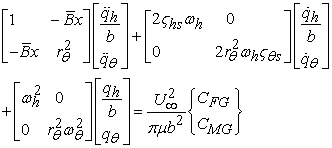(8)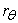is the radius of section gyration relative to the elastic axis and B is the coupling factor. With proper change in mass ratio, it is possible to examine the flutter condition in different heights.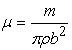Then, aeroelastic analysis is carried out using weak coupling. To do this, by having a mode shape and aerodynamic coefficient matrix of different frequencies, aerodynamic forces are calculated by Roger formulas and based on the reduced frequency.

Assuming that all the parameters in relation to the blades length are constant and regardless of blade twist, it can be said aerodynamic forces all nodes only depend on the mode shape of the blade. It can be said that aerodynamic forces of all nodes depend only on the mode shape of the blade. Thus: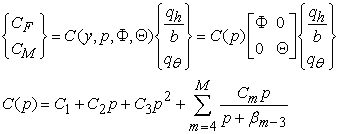(9)

In the above equation p is the aeroelastic frequency. So, in a nutshell, aeroelastic equation can be written as follows: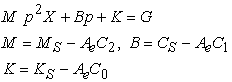(10)

G includes mass matrix and added damping and stiffness that increases the accuracy of the aerodynamic force. The above special value problem is resolved using state space and auxiliary state variable.(11)

Additionally, following formula is used to evaluate the effects of flutter, flexural and torsional movements in the conditions before, after and during flutter and compare with each other.(12)

### 9. Discussion and Calculations

The case is a row of tuned aeroelastic blades with standard tenth airfoil section located under the air flow.

In addition, considering Eq .4, we have: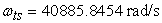In addition, the aerodynamic forces are calculated using the 'ANSYS / FLOTRAN' and the parameter value of Roger approximation was applied to aeroelastic equation. The natural frequency and speed of the one-end free blade flutter with no piezoelectric in the above conditions are shown in Table 1.

Moreover, natural flexural frequency in vacuum for Euler-Bernoulli beam was calculated using BEAM54 element, Timoshenko beam using BEAM44 element with the meshing of the beam section, natural torsional frequency of the blade with combining hardness elements COMBINE14, and the inertia moment elements MASS21, which approved the accuracy of the results presented in Table 1.

• Figure 1. flutter speed without piezoelectric
• Figure 2. flutter speed with piezoelectric
• Figure 3. Temporal simulation of curved airfoil blade vibration without piezoelectric, using the Timoshenko theory
• Figure 4. Temporal simulation of curved airfoil blade vibration with piezoelectric, using the Timoshenko theory

### 10. Conclusion

In this paper, flutter speed of the turbine blades was investigated. Blade equations that are obtained as one-end free beam model and the fluid dynamic forces were calculated. Then, flutter speed obtained by piezoelectric compared with the previous state. The results showed that piezoelectric optimal layout, flutter speed of gas turbines can be increased significantly.

### References

  Han S.M., Benaroya H., and Wei T., Dynamics of Transversly Vibrating Beams Using Four Engineering Theories, Journal of Sound and Vibration 225(5), 935-988, 1999. In article View Article  Lawrence C., Spyropoulos E., Reddy T.S.R., Unsteady Cascade Aerodynamic Response Using a Multiphysics Simulation Code, NASA/TM-209635, January 2000. In article View Article  Reddy T.S.R., Bakhle M.A., Trudell J.J., Mehmed O., Stefko G.L., LINFLUX-AE: A Turbomachinery Aeroelastic Code Based on a 3-D Linearized Euler Solver, NASA/TM-2004-212978, May 2004. In article View Article  Sadeghi M., Liu F., Coupled Fluid-Structure Simulation for Turbomachinery Blade Rows, 43rd AIAA Aerospace Sciences Meeting and Exhibit, AIAA 2005-0018, 10 - 13 Jan 2005, Reno, NV. In article View Article  Karadal F.M., Seber G., Sahin M., Nalbantoglu V., Yaman Y., State Space Representation of Smart Structures Under Unsteady Aerodynamic Loading, Ankara International Aerospace Conference, September 2007, Ankara, Turkey. In article View Article  S. Narayanana, V. Balamurugan, Finite element modelling of piezolaminated smart structures for active vibration control with distributed sensors and actuators, Sound and Vibration J., 262, 2003, pp. 529-562. In article View Article  S.X. Xu, T.S. Koko, Finite element analysis and design of actively controlled piezoelectric smart structures, Finite Element in Analysis and Design J., 40, 2004, pp. 241-262. In article View ArticleThis work is licensed under a Creative Commons Attribution 4.0 International License. To view a copy of this license, visit http://creativecommons.org/licenses/by/4.0/